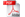ISSN 1842-4562 Member of DOAJJournal Home > Volume 12, Issue 1 - March 30, 2017

# JAQM Volume 12, Issue 1 - March 30, 2017Pdf Version

## Contents

This simulation study has been carried out to compare empirical type I error and test power of five tests namely Anom, Bartlett's, Levene's, Brown-Forsythe, Anom, and Conover tests to check homogeneity of variances. For this purpose, a comprehensive Monte Carlo Simulation study has been carried out for different number of groups (k=3, 4, 5, and 10), variance ratios (i.e: 1, 5, 10, 15, and 20), and sample size combinations (equal and unequal sample sizes) under normality assumption. Based on results of 50,000 simulation, it is observed that the best robust tests are the Anom and Bartlett’s tests even if studying with very small sample size (n=5) and a large number of group cases (k=10). They were followed by the Levene’s and Conover tests in general. But, both the Conover and Levene’s tests have been slightly negatively affected from increases in the number of groups when sample sizes were small (n≤20). On the other hand, since the Brown-Forsythe test did not give satisfactory results for any of the experimental conditions, it was concluded that the use of this test should not be preferred for checking homogeneity of variance assumption. As a result, since the Anom and Bartlett’s are robust tests for all experimental conditions, it is possible to propose to the researcher to use this test to check homogeneity of variances assumption prior to ANOVA and t-test.

Scales used to measure latent traits like behavioural attitudes are typically measured using classical statistical approaches. However, treating raw scores as interval scales present a fundamental problem when developing measures. To avoid these pitfalls human measurement instruments need to be constructed using Rasch analysis. The Rasch unidimensional model is currently the only method able to transform raw data into abstract equal-interval scales. The objective being for each personality dimension to have all items fit the Rasch model well, with the more endorsable items reliably preceding more difficult to endorse items in the direction of increasing levels of the underlying latent construct. Specifically, ensuring that all the items in each measured dimension manifests construct linearity and conjoint additivity. According to this view, if the data fit the model, then a scale with linearity and conjoint additivity will have been developed.

Biological systems cannot be understood by the analysis of single-type of dataset. So, integrative analysis has been considered as an essential tool to combine different types of datasets with the biological factors to improve biological knowledge. Several methods and approaches have been developed and updated time to time to integrate high-throughput datasets with the biological factors or supplementary variables. Among the several methods, multifactor analysis gives a rough idea about the association between gene to gene as well as gene to other biological factors. Therefore, using distance measure we aimed to develop an approach to find out the association between genes (manifest variables) and body weight gain (supplementary variable) more precisely. In order to conduct this study we used a secondary dataset. As multifactor analysis gives loadings of manifest variables and using loading plot we got approximate relationship between genes and body weight gain, therefore, we used distance formula to calculate distance between coordinates (loadings of first principal component as well as second principal component) of body weight gain and other gene(s). Taken together, we may conclude that distance measure gives better insight to find out the strength of association of two sets of transcriptomics datasets with the body weight gain compare to just observing loadings. The approach developed in this study is not only applicable in biological field but also can be applied any field of research where researcher wants to integrate several manifest variables with one or more supplementary variables.

High bank investment is important for supporting its funding center. But not all banks are active in investment banking, some banks its works limited on deposits and some banking service. In this paper, investment banking is a censored response variable at zero point. Therefore, the Tobit regression model (ToReg model) is considered more adaptable with censored response variable. But the ToReg model is no longer sufficient for data under study because (a) the distribution of investment banking shows skewness, (b) investment banking data have large gap between its small value and its large value, so this dataset has outlier values to overcome these problems by using the Tobit quantile regression model. Additionally, it gives us a complete description of the relationship between investment banking and a set of factors.